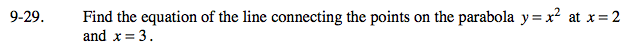### Home > CAAC > Chapter 16 > Lesson 16.9.1.2 > Problem9-29

9-29.1. Determine the (x, y) coordinates of the points of interest.
2. Calculate the slope between the two points.
3. Using one of the points and the slope, write the equation.